Technical Information Site of Power Supply Design

2022.03.09 motor

# Characteristics of Brushed DC Motors

Brushed DC Motor

Up until the previous article, we had explained the principles of brushed DC motors. Here we describe the basic characteristics of brushed DC motors.

## Characteristics of Brushed DC Motors

Put in simple terms, when a power supply voltage is applied to a motor, a current flows and the motor rotates; but the characteristics of the power supply voltage, the rotation rate, the torque, and other quantities are all interrelated. We explain these relations using an equivalent circuit and equations for a brushed motor.

●DC equation for the closed circuit: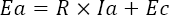＊Ea: Power supply voltage, R: Armature resistance,
Ia: Motor current, Ec: Motor induced voltage

The power supply voltage Ea is equal to the induced voltage Ec plus the product of the armature resistance R and the motor current Ia. The armature resistance has resistance components due to the windings and to the core. That the voltage is equal to resistance times current is precisely Ohm's law. The induced voltage is the voltage that occurs due to rotation of the motor (electricity generation), and is an additional voltage.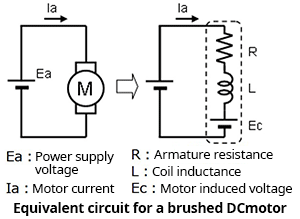●Motor induced voltage: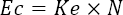＊Ec: Motor induced voltage, Ke: Power generation constant, N: Rotation rate

The motor induced voltage Ec is equal to the power generation constant Ke multiplied by the rotation rate N. Hence the induced voltage of the motor is proportional to the rotation rate.

●Motor torque: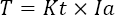＊T: Torque, Kt: Torque constant, Ia: Motor current

The motor torque T is the torque constant Kt multiplied by the motor current Ia. Hence the motor torque is proportional to the current.

●Relationship between rotation rate and torque: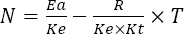The above-described equations for the induced voltage and torque of a motor are summarized, and the relationship between rotation rate N and torque T is illustrated below. Ke and Kt are constants, and so from the equations we can see that: 1) when a torque T is applied, the rotation rate N drops by a fixed amount; and, 2) when the torque T is a fixed value, the rotation rate N rises in proportion to the power supply voltage Ea.

The above relations are summarized in the diagram on the right. The torque-rotation rate (T-N) characteristic is such that when a torque is applied, the rotation rate falls by a fixed amount, that is, the relationship is inverse proportionality. And, when the power supply voltage is raised, the voltage applied to the motor rises, so that the rotation rate increases. The maximum torque occurs when the rotation rate is zero.

The torque-current (T-I) characteristic indicates that when a torque is applied, the motor current increases by a constant amount, that is, increases proportionally. At maximum torque, which occurs at zero rotation rate, the motor current is maximum.

When driving a motor, the driving conditions must be considered based on these relationships.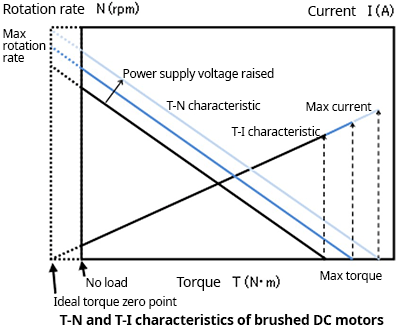## Motor Parameter Units

A number of motor parameters were introduced in the above explanations. The units in general use for these are as follows.

Rotation rate N: min-1, rpm, rad/s
Torque T: N･m
Power generation constant Ke: V･s/rad, V/rpm
Torque constant Kt: N･m/A

For some parameters, multiple units are used; as the units employed when converting electrical energy into mechanical energy, the N･m, rad/s and V･s/rad units indicated in boldface must be used consistently.

#### Key Points:

・When a torque is applied, the rotation rate drops by a constant amount.

・When the power supply voltage is raised, the rotation rate rises.

・When a torque is applied, the motor current increases by a constant amount.

・At maximum torque, which means zero rotation rate, the motor current is maximum.